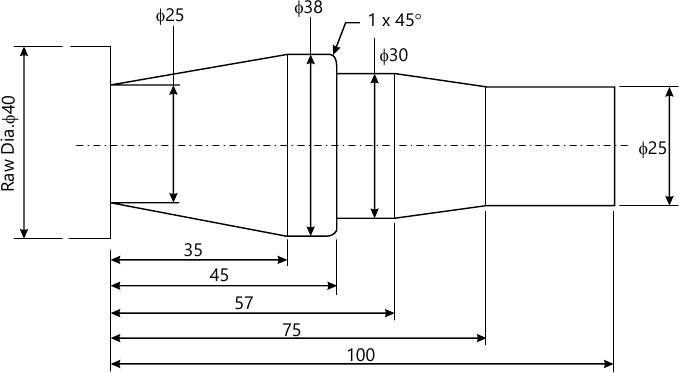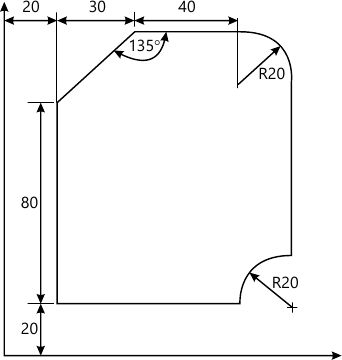MU Mechanical Engineering (Semester 7)
May 2015
Total marks: --
Total time: --
INSTRUCTIONS
(1) Assume appropriate data and state your reasons
(2) Marks are given to the right of every question
(3) Draw neat diagrams wherever necessary

1 (a) Write short note on concurrent engineering in product desig.
6 M
1 (b) Write short note on computer aided process planing (CAPP).
7 M
1 (c) Plot the Bezier curve having endpoints P0(1, 3) and P3(7, 2). The other control points are P1(5, 6) and P2 (6, 0). Plot for values for u=0, 0.1, 0.2, ... 1, if the characteristic polygon is drawn in the sequence P0 - P1 - P2, P3.
7 M

2 (a) Write a part program using G' and M codes for finishing a forged component as shown in Figure 1. Assume the speed and feed on the turning cente as 500 rpm and 0.3 mm/rev respectively. Assume suitable data if necessary.10 M
2 (b) Consider a triangle ABC having coordinates A(5,5) B(8,5) and C(5,10). Determine the new vertex position if.
i) The triangle is rotated by 60°, anticlockwise about the vertex A.
ii) The triangle is scale by 2 times in X direction and 3 times in Y direction about vertex A.
10 M

Write short note on following:
3 (a) Adaptive control in manufacturing.
8 M
3 (b) Artificial Intelligence in Computer Aided Process Planning (CAPP).
6 M
3 (c) Socio-techno-economic aspects with respect to Computer Integrated Manufacturing (CIM).
6 M

Write short note on:
4 (a) (i) Essential of Computer Aided Design workstations and its functions.
5 M
4 (a) (ii) Visual realism.
5 M
4 (b) Write a complete APT program (geometric and motion commands) to machine the outline of the geometry as shown in Figure 2. The component is 5 mm tick. The milling tool used is 5 mm in diameter. Consider spindle speed as 1000 rpm and feed as 0.3 mm/rev. Assume suitable data if necessary.5 M

5 (a) Write a program in object oriented language for 2D transformation which include functions for the following operations: (i) rotation @y-axis (ii) translation in x-direction.
10 M
Write short note on:
5 (b) (i) Reverse engineering and data capture techniques.
5 M
5 (b) (ii) Green Manufacturing.
5 M

6 (a) Explain with block diagram Computer Aided Quality Control (CAQC).
10 M
Write short note on:
6 (b) (i) Light and shade ray tracing.
5 M
6 (b) (ii) Design for assembly and disassembly.
5 M

Write short note on:
7 (a) Automated material handling and storage system
6 M
7 (b) Flexible manufacturing systems (FMS).
6 M
7 (c) Feature recognition and design by feature.
8 M

More question papers from CAD / CAM / CIM